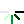Python3之深浅copy## ☞几个术语的解释：

1，变量：是一个系统表的元素，拥有指向对象的连接空间

2，对象：被分配的一块内存，存储其所代表的值

3，引用：是自动形成的从变量到对象的指针

4，注意：类型（int类型，long类型(python3已去除long类型，只剩下int类型的数据)）属于对象，不是变量

5，不可变对象：一旦创建就不可修改的对象，包括字符串、元组、数字

6，可变对象：可以修改的对象，包括列表、字典。

## ☞应用的范围：

1，切片可以应用于：列表、元组、字符串，但不能应用于字典。

2，深浅拷贝，既可应用序列（列表、元组、字符串），也可应用字典。

## ☞深浅拷贝的作用：

1，减少内存的使用

2，以后在做数据的清洗、修改或者入库的时候，对原数据进行复制一份，以防数据修改之后，找不到原数据。

## ☞对于不可变对象的深浅拷贝：

``````import copy  # 使用拷贝需要导入copy模块

a=(1,2,3)
print("=====第一种=号浅拷贝=====")
b=a
print(a)
print(b)
print(id(a))
print(id(b))
print("=====另一种copy浅拷贝===")
b=copy.copy(a)
print(a)
print(b)
print(id(a))
print(id(b))
print("=====深拷贝=====")
b=copy.deepcopy(a)
print(a)
print(b)
print(id(a))
print(id(b))
# 结果如下：
=====浅拷贝=====
(1, 2, 3)
(1, 2, 3)
2814522335952
2814522335952
=====另一种浅拷贝===
(1, 2, 3)
(1, 2, 3)
2814522335952
2814522335952
=====深拷贝=====
(1, 2, 3)
(1, 2, 3)
2814522335952
2814522335952
``````

## ☞对于可变对象深浅拷贝:

=浅拷贝：值相等，地址相等

copy浅拷贝：值相等，地址不相等

deepcopy深拷贝：值相等，地址不相等

``````import copy  # 使用拷贝需要导入copy模块

a=[1,2,3]
print("=====第一种=号浅拷贝=====")
b=a
print(a)
print(b)
print(id(a))
print(id(b))
print("=====另一种copy浅拷贝===")
b=copy.copy(a)
print(a)
print(b)
print(id(a))
print(id(b))
print("=====深拷贝=====")
b=copy.deepcopy(a)
print(a)
print(b)
print(id(a))
print(id(b))
#结果如下：
=====浅拷贝=====
[1, 2, 3]
[1, 2, 3]
2007696321544
2007696321544
=====另一种copy浅拷贝===
[1, 2, 3]
[1, 2, 3]
2007696321544
2007695909960
=====深拷贝=====
[1, 2, 3]
[1, 2, 3]
2007696321544
2007696319560
``````

## ☞总结：

1，深浅拷贝都是对源对象的复制，占用不同的内存空间。

2，不可变类型的对象，对于深浅拷贝毫无影响，最终的地址值和值都是相等的。

3，可变类型：

=浅拷贝： 值相等，地址相等

copy浅拷贝：值相等，地址不相等

deepcopy深拷贝：值相等，地址不相等

## ☞参考

转载请注明: My blog Python3之深浅copy

目录